Project tutorial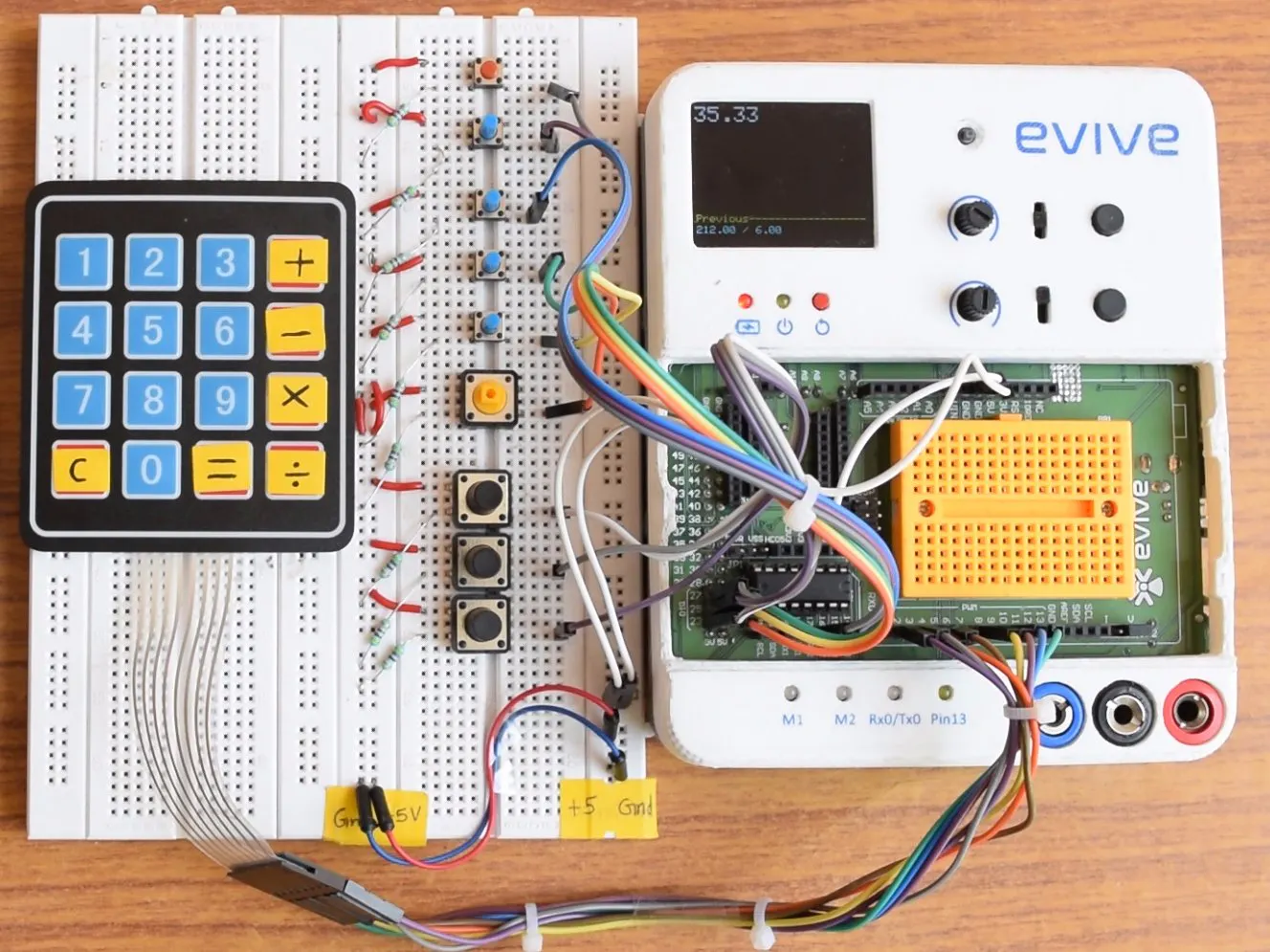Its a Scientific Calculator (4 decimal accuracy) with functions like +, -, *, %, Sin, Cos, Tan, arcSin, arcCos, aTan, log made using evive.

• 9,881 views
• 18 respects

Components and supplies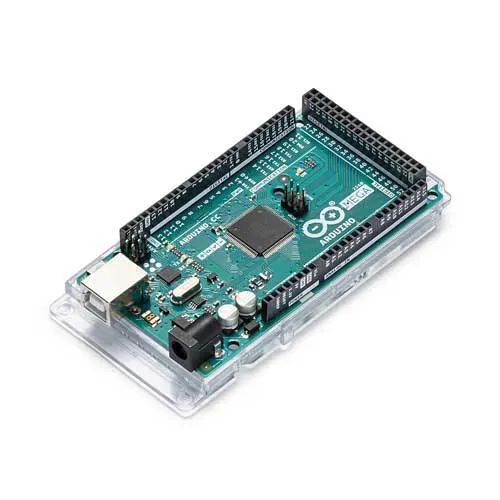Arduino Mega 2560 We have used evive. Its an Arduino MEGA powered embedded platform. http://evive.cc
×1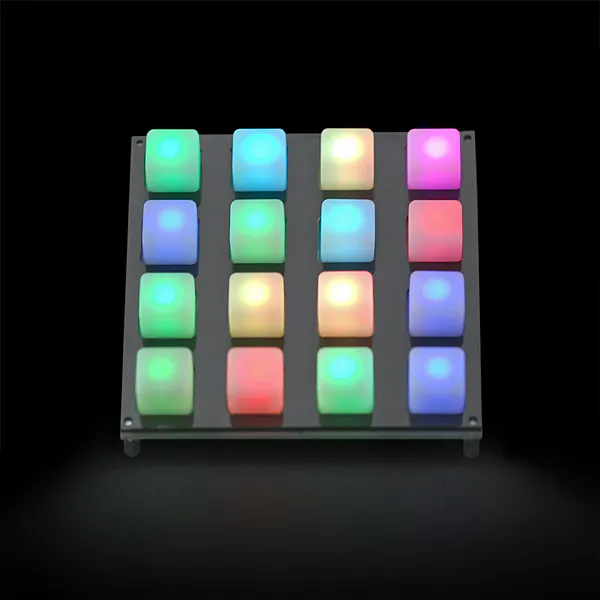SparkFun Button Pad 4x4 - LED Compatible We have used similar 4X4 Keypad like this one http://www.ebay.in/itm/151964034460?aff_source=Sok-Goog
×1SparkFun Pushbutton switch 12mm Similar to this, many different types of switches were used for different functions to make it more user friendly
×9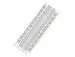×1Resistor 1k ohm
×9Jumper wires (generic)
×20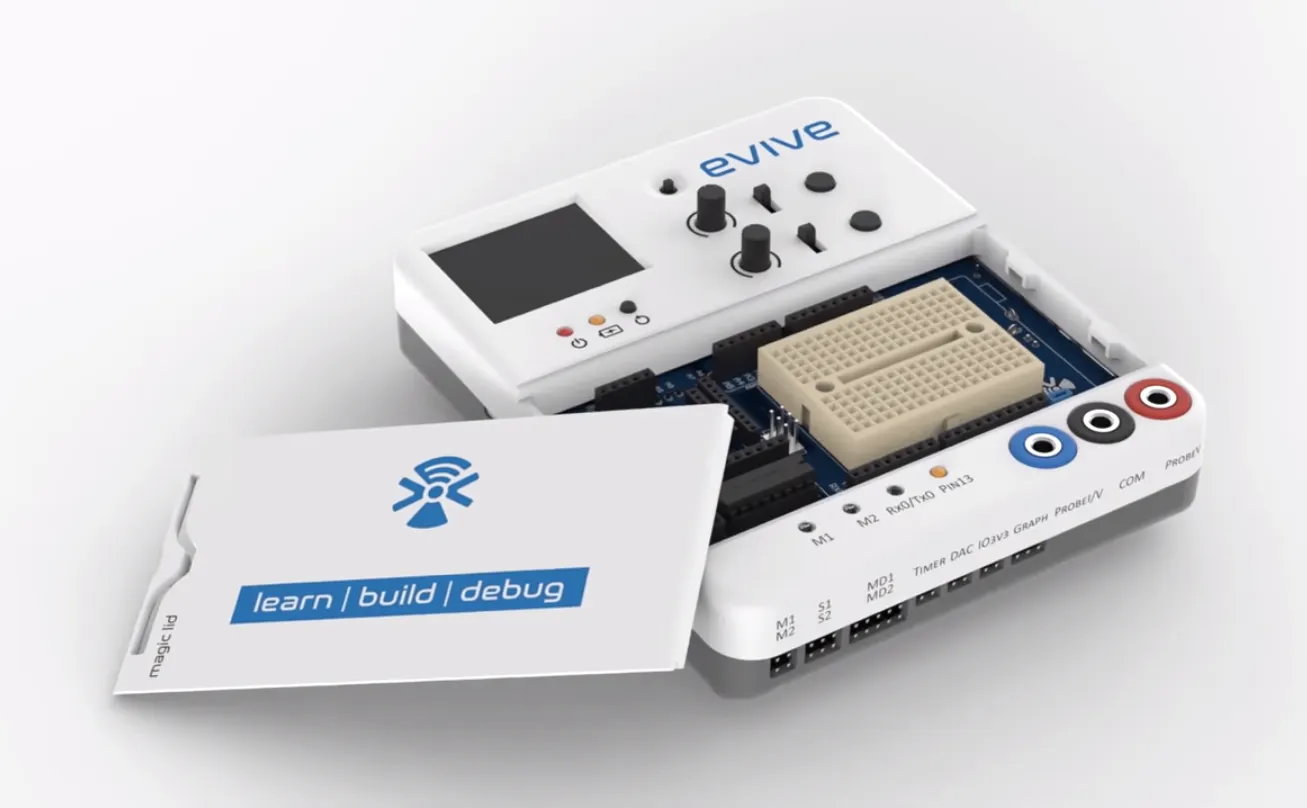STEMpedia evive
×1

Apps and online services

We have built a Scientific calculator having lots of functions like

• Basic algebraic operations (+, -, *, %)
• Trigonometric functions (SINe, COSine, TANgent)
• Inverse trigonometric functions (arcSin, arcCos, arcTan)
• Logarithmic function
• Square root.

An arduino sketch is made to implement this. It calculates accurately upto six decimal places and display upto four decimal place.

Step 1: Components Required

The evive's colorful display makes it a bit ahead of other arduino calculators.

Components used :

• Pushbuttons of different colors (or different types to make it more user friendly)
• Dupont (Jumper) Cables
• Resistors (for pull-down) (~1kOhm)
• Write-able tape

Step 2: Making the Simple Calculator

Firstly we will make a simple calculator that does simple operations like addition, subtraction, multiplication and division. We have used the A, B, C, D keys for the above operations respectively. For the 'equal to' operation we have used '#' key and for 'clear the screen' we have used '*' key in the keypad. In the attached image, connections for keypad are mentioned.

For the coding part we have taken help from the already build code at the arduino site-

Rest is just tweaking it to run by changing the pins etc. We have modified the code for 1.8" TFT (ST7735R).

But this was very simple!! Lets go ahead

For both our input numbers the algorithm is basically the same.

Let the first number be a double named 'first'. We initialize it to be zero.

We make a Boolean 'isDecimal' and initialize it as false. This means that unless the decimal point is given as an input, the number is not a decimal.

Last, declare a float 'decimals' and set it as 10.0. We will use it for keeping a counter of our place after the decimal point.

Now if isDecimal is false, it means the number is not yet a decimal. Suppose you are storing your input number from the keypad as key.

You just need to update first=first*10+key.

But if isDecimal is true, the number is a decimal. You need to now update as

first=first+key/decimals, and decimals=decimals*10.

We keep repeating the above steps until the input for some operation is detected. Then we similarly detect the second number. Using the knowledge of the operation called, we operate the numbers and print the result when '=' is detected.

Remember to restate the values of decimals=10.0 and isDecimal=false after detection for a number is complete.

Step 4: Preparing the Breadboard Connections for the Other Functions

Now, we have to add more buttons for the scientific functionality. We have used some colorful buttons as shown in figure. Now assign them accordingly. Take care to assign similar buttons to similar functions.

Since evive uses Arduino Mega, we have used following pins:

• sine=Pin25
• cosine=Pin27
• tangent=Pin23
• log=Pin22
• arcsin=Pin24
• arccos=Pin26
• arctan=Pin14
• root=Pin15

Step 5: Printing the Calculation Log

We have printed the calculation log also. In the bottom of the screen you can print the calculation log by just remembering the first and the second numbers of the previous calculation.

Step 6: Building the Functions for other Operators

First try making the code for any one function (for example, sine function) using the inbuilt function 'sin(val)' of the arduino ide. Arduino ide also has pre-installed functions of cos, tan, inverse sin, inverse cos, inverse tan, log, square root. We have also added one more button for the decimal.

You can view the following image for reference for making different functions.

All the codes can be downloaded from GitHub. The code is well documented and self explanatory.

Step 7: Conclusion and Testing

The calculator works well!! We have also used evive's inbuilt buzzer to provide audio feedback in form of a beep while someone presses any button or switch. You can design the calculator which prints the results on the tft screen of the evive. Use different colors and sizes of text to decorate it. We can align the operants and the functions to make the appearance better.

Add more functions and dig harder to make it more complex :P

You can check more features and techSpecs of evive to make it more advanced calculator. Watch the video of evive here

Code

Github
https://github.com/evivetoolkit/eviveProjects/tree/master/scientificCalculator

DIY piano using scratch and evive

Project tutorial by evive: an opensource embedded platoform

• 5,389 views
• 10 respects

Plant monitoring and watering system using Evive

Project tutorial by Team evive: an opensource embedded platoform

• 20,842 views
• 1 comment
• 39 respects

Ultrasonic Obstacle Avoiding Robot Using Evive

Project tutorial by Team evive: transform your ideas to reality!

• 15,987 views
• 17 respects

8 x 8 x 8 LED Cube Powered by an Arduino Mega

Project tutorial by Doug Domke

• 18,248 views
• 26 respects

Remote Controlled Shopping Cart Using evive

Project tutorial by STEMpedia

• 2,728 views